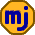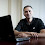## Monday, October 7, 2019

### Correlation

Smart people who analyze different investment strategies often talk about correlations. Investments have correlations that are high, low, positive, or negative. This can all sound impressive, but as I’ll show, any conclusions we draw based on correlations can be suspect.

In the investment world, correlation is a measure of how asset returns move together. A positive correlation means two assets tend to give good returns together and bad returns together. A negative correlation means they tend to move in opposite directions. A zero correlation means the direction of one investment doesn’t tell you anything about the direction of the other investment.

It’s impossible to know the correlation of two investments exactly. All you can do is measure their correlation over a period of time. We then just assume the correlation will remain the same into the future.

To show the problem with this approach, I simulated two streams of monthly investment returns. The distributions I chose had zero correlation. Then I measured the rolling 10-year correlations of the two investments. Here are the results.

As we can see, the measured correlations aren’t particularly close to zero much of the time. The range was -11% to +24%. The fact that the correlations tended to be positive was just a coincidence; when I ran it a few more times, sometimes the correlations tended to be negative.

So, even though the correlation of the distributions I used was zero, the measured correlations from sequences of outputs varied considerably. So, analysts combing through investment returns could easily think the correlation between two investments is 20% when it is really zero.

Even more disturbing is that much mathematical analysis of investing assumes that returns follow the normal distribution (technically the lognormal distribution). However, there is strong evidence that the tails of return distributions follow power laws rather than the normal curve. This means that the standard deviation is infinite, and that correlations (in the way they are usually calculated) don’t exist.

These unsettling facts are the main reason why an analysis might calculate the optimum leverage for a portfolio to be 300%, when the real answer is more like 0% or 25%.

This doesn’t mean that any investment analysis that discusses correlations is automatically wrong. All mathematical analyses use models, and all models fail to match the real world in one way or another. The challenge is to figure out when the math gives the right answers and when it doesn’t.

Only a small minority of investors are comfortable using the math described in this article. However, among those who are comfortable with this math, only a small minority understand the errors in the models they use and how they cast doubt on their conclusions.

1.Your point about not trusting too much in measured correlations, or any conclusions drawn from them, is very important. However, I’d hope anyone in financial or risk planning faced with a measured correlation of 25% would interpret it as Occam’s-Razor equivalent to 0 anyway. After all, it implies only 6% of observed variance (25% squared) is explained by a hypothesized linear relationship.

1.@Martin: In my experience, people who understand the limits of the mathematical tools they use are rare. It's one thing to understand that measured correlations are inaccurate, but quite another to understand the potential impact of this inaccuracy on any conclusions drawn from some mathematical analysis. Even worse is the tendency to declare inconvenient model inaccuracies as unimportant. Even some Nobel Prize winners think that return autocorrelations aren't a concern.

2.I don't disagree, but I think you're overstating the case. In my field - risk management - at least, the one lesson that has been learnt well in the past decade (of the multiple lessons that *should* have been learned...) is to stress-test correlation breakdown. So for instance conclusions are tested when measured correlations are replaced by 1 or 0. And the economic capital, broadly speaking, needs to cover that - the analog of your leverage point.

I do think many are still too wedded to the mathematics, and do the "stress testing" (or whatever) formulaically rather than thoughtfully. The point should be not what if instead of r=... you try r=..., or instead of distribution X you try X'. It should be thoughtfully challenging what fundamental macro and other assumptions you're making and their implications. Too few do that, I fully agree.

3.@Martin: I don't believe I'm overstating the case. Mathematical tools were horribly misused to calculate risk levels of mortgage-backed securities leading up to the credit crisis. Monte Carlo simulators used to stress-test individual financial plans routinely ignore long-term momentum effects in bonds. It's not hard to find people using mathematical tools without understanding the implicit assumptions they are making.

2.Michael,

What are your thoughts on Nassim Taleb's philosophy towards investing? I think he believes the vast majority of investors can't protect for tail risk so they should dramatically reduce exposure to risky assets which is pretty much everything except GICs and very short term government bonds.

1.@Larry C.: Just about every asset has more tail risk than standard mathematical models allow, so I'm with Taleb there. I've read much of Taleb's writing and have seen that he has bet against investments with high exposure to tail risk. I've never read anything from him saying people should avoid stocks, but if he did, I disagree. Just don't be in stocks with any money you'll need within 5 years.

3.If you're interested, forward to 1:30.

1.